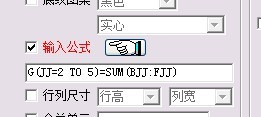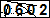公式代码: G(JJ=3 to 17)=SUM(DJJ:FJJ)  说明: 用公式计算：D3+E3+F3的和放到单元格G3中                       ↓             D17+E17+F17的和放到单元格G17中 公式代码: (JJ=B TO F)6=AVERAGE(JJ2:JJ5) 说明: 用公式计算：B2至B5的平均值放到单元格B6中                        ↓             F2至F5的平均值放到单元格F6中 公式代码: (JJ=B TO F)7=MAX(JJ2:JJ5) 说明: 用公式计算：B2至B5的最大值放到单元格B7中                        ↓             F2至F5的最大值放到单元格F7中 公式代码: (JJ=B TO F)8=MIN(JJ2:JJ5) 说明: 用公式计算：B2至B5的最小值放到单元格B8中                        ↓             F2至F5的最小值放到单元格F8中 公式代码: A,2=MAX(E3:E17) 说明: 用公式计算：E3至E17的最大值放到单元格A2中 公式代码: A,3=MIN(E3:E17) 说明: 用公式计算：E3至E17的最小值放到单元格A3中 公式代码: A,4=AVERAGE(E3:E17) 说明: 用公式计算：E3至E17的平均值放到单元格A4中 公式代码: A,4=AVERAGE(E3:E17) 说明: 用公式计算：E3至E17的平均值放到单元格A4中 公式代码: D,2=(TODAY()-C2)/365 说明: 单元格(D,2)的计算代码为:=(TODAY()-C2)/365 公式代码: G,2=REPLACE(F2,5,1,86) 说明: 单元格(G,2)的计算代码为:=REPLACE(F2,5,1,86) 公式代码: H,2=IF(AND(D2>=40,B2="男"),"是","否") 说明: 单元格(H,2)的计算代码为:=IF(AND(D2>=40,B2="男"),"是","否") 公式代码: B,2=COUNTIF(SHEET1!B2:B3,"男") 说明: 单元格(B,2)的计算代码为:=COUNTIF(SHEET1!B2:B3,"男") 公式代码: B,3=COUNTIF(SHEET1!D2:D3,">40") 说明: 单元格(B,3)的计算代码为:=COUNTIF(SHEET1!D2:D3,">40")

• 发表评论
• 用户：  验证码：Keywords: 考试系统 考试系统